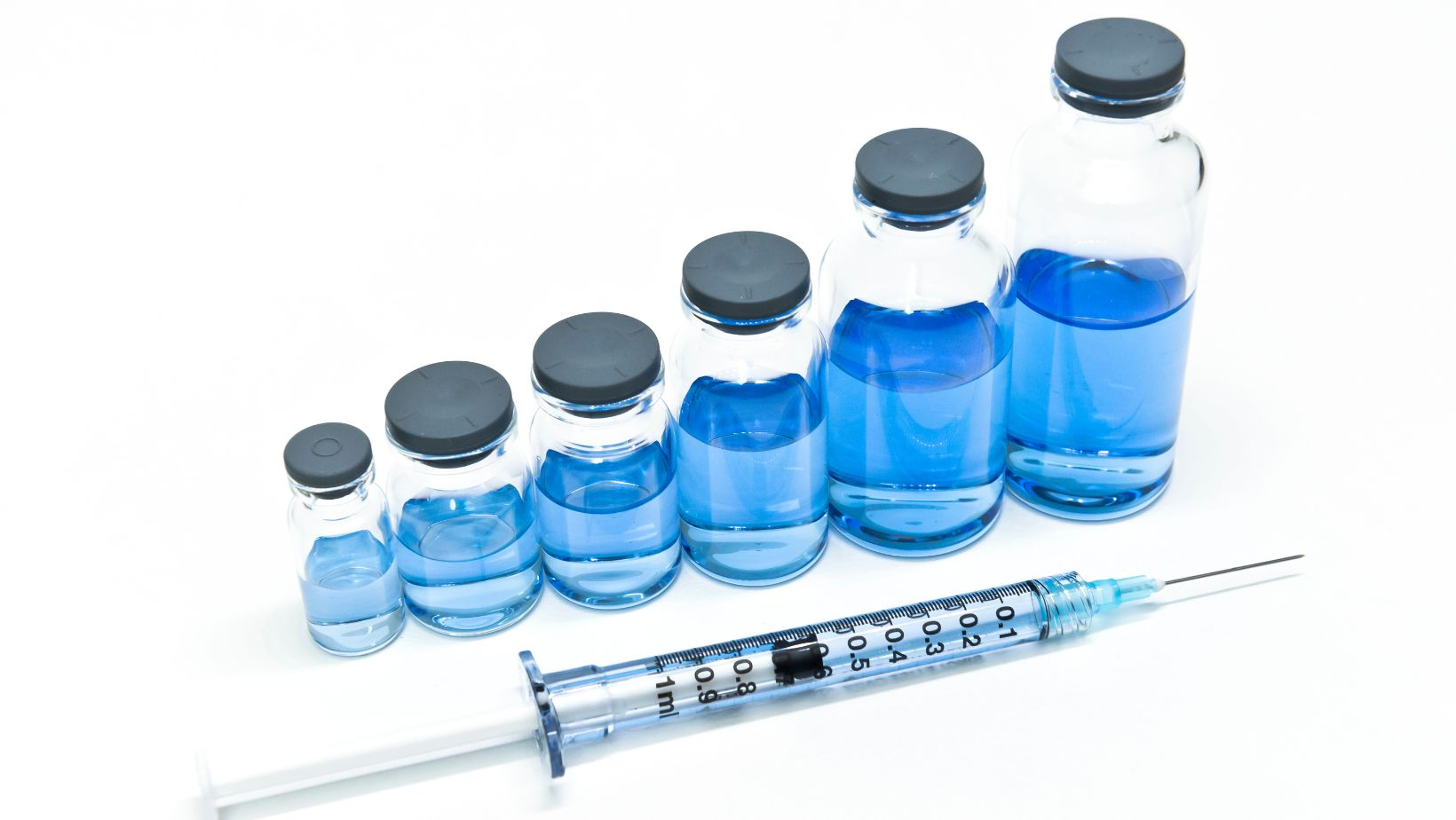# Basic Conversion Guide on How Many Ul is in a Ml## How Many Ul is in a Ml

Wondering how many ul (microliters) are in a ml (milliliter)? Well, you’ve come to the right place! The conversion between these two units of volume is straightforward and can be easily understood. Let me break it down for you.

To put it simply, there are 1000 microliters in one milliliter. Both ul and ml are commonly used measurements in scientific experiments and medical applications. Understanding this conversion is essential for accurate measurement and dosage calculations.

So, whether you’re conducting laboratory research or administering medication, knowing that 1 ml equals 1000 ul will help you ensure precise measurements. Now that we have cleared up the confusion surrounding this topic, let’s dive deeper into the fascinating world of units of volume!

## Understanding the Conversion: UL to ML

### UL to ML Conversion Explained

When it comes to measuring small volumes of liquid, you may often come across two common units: microliters (UL) and milliliters (ML). Understanding the conversion between these two units is essential for accurate measurements in scientific experiments, medical dosages, or even in cooking recipes.

To grasp the concept of converting UL to ML, we must first understand their relationship. One milliliter (ML) is equal to one thousand microliters (UL), making it a larger unit of measurement. This means that if you have 5000 microliters, it would be equivalent to 5 milliliters.

### Converting UL to ML: A Step-by-Step Guide

Converting microliters (UL) to milliliters (ML) may sound daunting at first, but with a simple step-by-step approach, you’ll find it easier than you think:

1. Identify the value in microliters: Determine the quantity you want to convert from UL to ML.
2. Divide by 1000: Since there are 1000 microliters in one milliliter, divide the number of microliters by 1000.
3. Obtain the result: The quotient obtained from dividing will give you the value in milliliters.

For example, let’s say you have 2500 microliters that need conversion into milliliters:

2500 UL ÷ 1000 = 2.5 ML

So, your original measurement of 2500 microliters equals 2.5 milliliters after conversion.

### Common Conversions: UL to ML

Knowing some common conversions from microliters (UL) to milliliters (ML) can be useful in various scenarios. Here are a few examples to give you an idea:

• 500 UL is equal to 0.5 ML
• 1000 UL is equal to 1 ML
• 7500 UL is equal to 7.5 ML

Having these conversions at your fingertips can save time and help ensure accurate measurements in your scientific or culinary endeavors.

Remember, when dealing with small volumes of liquid, precision matters. So understanding the conversion from microliters (UL) to milliliters (ML) enables you to confidently measure and work with liquids in a more efficient and precise manner.

Now that we’ve covered the essentials of converting UL to ML, let’s explore other intriguing aspects of liquid measurement and conversion methods.

## The Definition of a Microliter (UL)

In this section, I’ll provide you with an overview of what a microliter (UL) is and how it relates to milliliters (ML).

### Understanding the Microliter Measurement

A microliter, often abbreviated as UL, is a unit of volume measurement in the metric system. It represents one millionth of a liter or one thousandth of a milliliter. This tiny unit is commonly used in scientific research, medical laboratories, and pharmaceutical industries for precise measurements.

### Converting Microliters to Milliliters

To convert microliters to milliliters, we need to remember that there are 1,000 microliters in one milliliter. Here’s the formula:

Milliliters (ML) = Microliters (UL) / 1000

For example, if we have 5000 microliters, we can convert it to milliliters using the formula:

Milliliters (ML) = 5000 UL / 1000 = 5 ML

## Practical Applications

Microliters are particularly useful when dealing with very small volumes of liquids. They are commonly used in applications such as:

• Pipetting small amounts of reagents in biochemical experiments.
• Measuring precise quantities of medication in pharmaceutical preparations.
• Analyzing DNA or protein samples where accuracy is paramount.

It’s worth noting that even though microliters and milliliters may seem similar due to their small size, they serve different purposes based on the required precision.

Overall, understanding the definition and conversion between microliters and millilitres allows us to work effectively within various scientific fields where accurate liquid measurements are crucial.

Now that we have covered the basics of what a microliter is and its relation to millilitres, let’s move on to the next section where we explore further measurements and conversions.

Paige is a loving wife and an excellent chef. She owns Justalittlebite.com, a website that shares recipes and cooking tips. Paige loves spending time in the kitchen, where she can experiment with new flavors and techniques. Her husband appreciates her delicious cooking, as do her many friends and followers online. When she's not in the kitchen, Paige enjoys spending time with her family and friends.